# GSEB Solutions Class 6 Maths Chapter 7 Fractions Ex 7.4

Gujarat Board GSEB Textbook Solutions Class 6 Maths Chapter 7 Fractions Ex 7.4 Textbook Questions and Answers.

## Gujarat Board Textbook Solutions Class 6 Maths Chapter 7 Fractions Ex 7.4

Question 1.
Write shaded portion as fraction. Arrange them in ascending and descending order using correct sign ‘<‘, ‘=’ ‘>’between the fractions: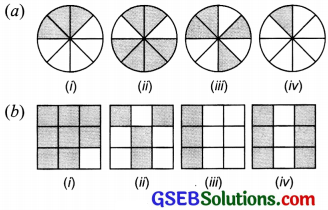(c) (i) show $$\frac { 2 }{ 6 }$$, $$\frac { 4 }{ 6 }$$, $$\frac { 8 }{ 6 }$$ and $$\frac { 8 }{ 6 }$$ on the number line.

(ii) Put appropriate signs between the fractions given.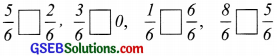Solution:
(a) Figure (i) represents fraction = $$\frac { 3 }{ 8 }$$
Figure (ii) represents fraction = $$\frac { 6 }{ 8 }$$
Figure (iii) represents fraction = $$\frac { 4 }{ 8 }$$
Figure (iv) represents fraction = $$\frac { 1 }{ 8 }$$
These are like fractions. So we arrange them in order to their numerators.
Ascending order: $$\frac { 1 }{ 8 }$$ < $$\frac { 3 }{ 8 }$$ < $$\frac { 4 }{ 8 }$$ < $$\frac { 6 }{ 8 }$$ Descending order: $$\frac { 6 }{ 8 }$$ > $$\frac { 4 }{ 8 }$$ > $$\frac { 3 }{ 8 }$$ > $$\frac { 1 }{ 8 }$$

(b) Figure (i) represents a fraction = $$\frac { 8 }{ 9 }$$
Figure (ii) represents a fraction = $$\frac { 4 }{ 9 }$$
Figure (iii) represents a fraction = $$\frac { 3 }{ 9 }$$
Figure (iv) represents a fraction = $$\frac { 6 }{ 9 }$$
The fractions are like fractions with different numerators.
Ascending order: $$\frac { 3 }{ 9 }$$< $$\frac { 4 }{ 9 }$$ < $$\frac { 6 }{ 9 }$$ < $$\frac { 8 }{ 9 }$$ Descending order: $$\frac { 8 }{ 9 }$$ > $$\frac { 6 }{ 9 }$$ > $$\frac { 4 }{ 9 }$$ > $$\frac { 3 }{ 9 }$$

(c) (i) Given fractions are: $$\frac { 2 }{ 6 }$$, $$\frac { 4 }{ 6 }$$, $$\frac { 8 }{ 6 }$$ and $$\frac { 6 }{ 6 }$$
We can have: $$\frac { 2 }{ 6 }$$ < $$\frac { 4 }{ 6 }$$ < $$\frac { 8 }{ 6 }$$ < $$\frac { 6 }{ 6 }$$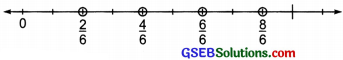(ii) We have: $$\frac { 5 }{ 6 }$$ > $$\frac { 2 }{ 6 }$$; $$\frac { 3 }{ 6 }$$ > 0
$$\frac { 6 }{ 6 }$$ < $$\frac { 1 }{ 6 }$$; $$\frac { 8 }{ 6 }$$ < $$\frac { 5 }{ 6 }$$Question 2.
Compare the fractions and put an appropriate sign.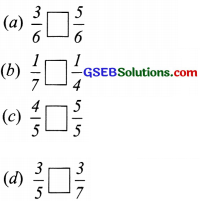Solution:
(a) Here, we have ‘like fractions’ so we compare them by their numerators only.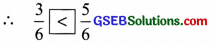(b) Here, we have ‘unlike fractions’ with same numerators, so we compare them with their denominators only.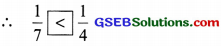(c) Like fractions, so we compare by their numerators only.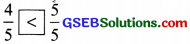(d) ‘Unlike fractions’ with same numerators so we compare them by their denominators, only.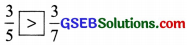Question 3.
Make five more such pairs and put appropriate signs.
Solution:
Five more examples can be given as below: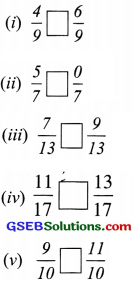After writing the appropriate signs, we have: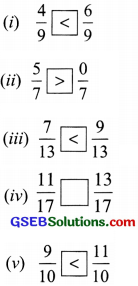Question 4.
Look at the figures and write ‘<‘ ‘>’or ‘=’ between the given pairs of fractions.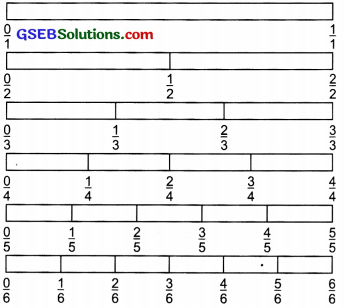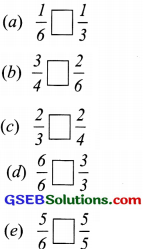Make five more such problems and solve them with your friends.
Solution:
(a) In the figure, $$\frac { 1 }{ 6 }$$ is on the left of $$\frac { 1 }{ 3 }$$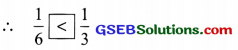(b) In the figure, $$\frac { 3 }{ 4 }$$ lies on the right of $$\frac { 2 }{ 6 }$$.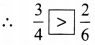(c) In the figure, $$\frac { 2 }{ 3 }$$ is on the right of $$\frac { 2 }{ 4 }$$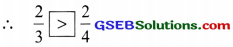(d) In the figurc, $$\frac { 6 }{ 6 }$$ is on the same point as of $$\frac { 3 }{ 3 }$$.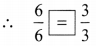(e) In the figure, $$\frac { 5 }{ 6 }$$ is at the same point as $$\frac { 5 }{ 5 }$$.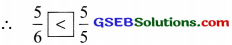Five more examples can be given as below:
Look at the previous figure and write ‘<‘, ‘>‘
or ‘=’ between the following pairs of fractions: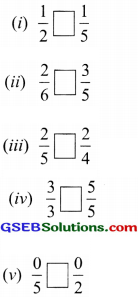After writing the appropriate signs, we have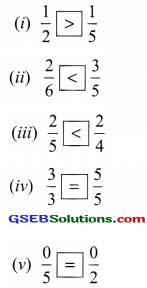Question 5.
How quickly can you do this? Fill appropriate sign. (‘<‘, ‘=’, ‘>’)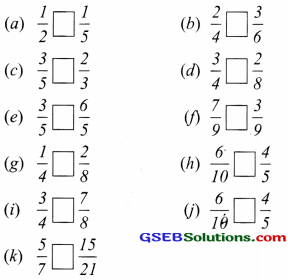Solution:
We use cross-product method for quicker calculations.
We have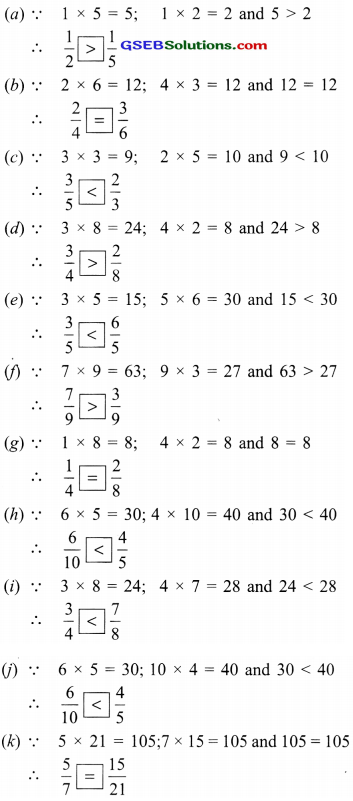Question 6.
The following fractions represent just three different numbers. Separate them into three groups of equivalent fi-actions, by changing each one to its simplest form.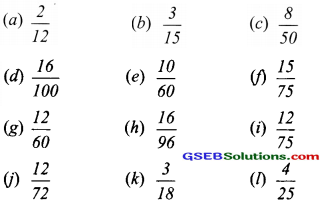Solution: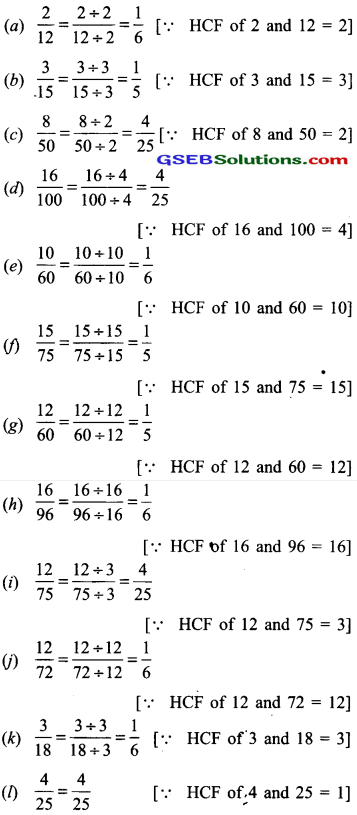Grouping the simplest forms, we have: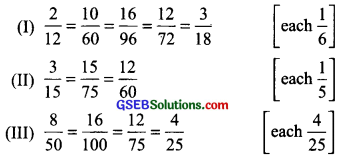Question 7.
Find answers to the following. Write and indicare how you solved them,
(a) Is $$\frac { 5 }{ 9 }$$ equal to $$\frac { 4 }{ 5 }$$ ?
(b) Is $$\frac { 9 }{ 16 }$$ equal to $$\frac { 5 }{ 9 }$$ ?
(c) Is $$\frac { 4 }{ 5 }$$ equal to $$\frac { 16 }{ 20 }$$ ?
(d) Is $$\frac { 1 }{ 15 }$$ equal to $$\frac { 4 }{ 30 }$$ ?
Solution:
(a) By cross product, we have
5 x 5 = 25 and 9 x 4 = 36
25 ≠ 36 i.e 5 x 5 ≠ 9 x 4
$$\frac { 5 }{ 9 }$$ ≠ $$\frac { 4 }{ 5 }$$ i.e $$\frac { 5 }{ 9 }$$, is not equal to $$\frac { 2 }{ 7 }$$.

(b) y cross product, we have
9 x 9 = 81 and 16 x 5 = 80
81 ≠ 80,i.e.9 x 9 ≠ 16 x 5
$$\frac { 9 }{ 16 }$$ ≠ $$\frac { 5 }{ 9 }$$, i.e. $$\frac { 9 }{ 16 }$$ is not equal to $$\frac { 5 }{ 9 }$$.

(c) By cross product, we have
4 x 20 = 80 and 5 x 16 = 80
4 x 20 = 5 x 16
$$\frac { 4 }{ 5 }$$ = $$\frac { 16 }{ 20 }$$, i.e. $$\frac { 16 }{ 20 }$$ and $$\frac { 4 }{ 5 }$$ are equal.

(d) By cross product, we have
1 x 30 = 30 and 4 x 15 = 60
30 ≠ 60, i.e. 1 x 30 ≠ 4 x 15
$$\frac { 1 }{ 5 }$$ ≠ $$\frac { 4 }{ 30 }$$Question 8.
Ila read 25 pages of a book containing 100 pages. Lauta read $$\frac { 2 }{ 5 }$$ of the same book. Who read less?
Solution:
Fraction of book read by Illa = $$\frac { 25 }{ 100 }$$
$$\frac{25 \div 25}{100 \div 25}$$ = $$\frac { 1 }{ 4 }$$
Fraction of book read by Lauta = $$\frac { 2 }{ 5 }$$
To compare $$\frac { 1 }{ 4 }$$ and $$\frac { 2 }{ 5 }$$ we have, 1 x 5 = 5;
4 x 2 = 8 and 5 < 8
$$\frac { 1 }{ 4 }$$ < $$\frac { 2 }{ 5 }$$ Thus Ila read less. Question 9. Rafiq exercised for $$\frac { 3 }{ 6 }$$ of an hour, while Rohit exercised for $$\frac { 3 }{ 4 }$$ of an hour Who exercised for a longer time? Solution: Fraction of an hour for which Rafiq exercised = $$\frac { 3 }{ 6 }$$ Fraction of an hour for which Rohit exercised = $$\frac { 3 }{ 4 }$$ To compare $$\frac { 3 }{ 6 }$$ and $$\frac { 3 }{ 4 }$$ we have: For ‘unlike fractions’ with same numerator, greator fraction has lesser denominator. $$\frac { 3 }{ 4 }$$ > $$\frac { 3 }{ 6 }$$
Rohit exercised for a longer period.Question 10.
In a class A of 25 students, 20 passed in first class; in another class B of 30 students, 24 passed in first class. In which class was a greater fraction of students getting first class?
Solution:
In class A, fraction of students who got 1st division
= $$\frac { 20 }{ 25 }$$ = $$\frac{20+5}{25 \div 5}$$ = $$\frac { 4 }{ 5 }$$ ………….(i)
In class B, fraction of students who got 1st division
$$\frac { 24 }{ 25 }$$ = $$\frac{24 \div 6}{30 \div 6}$$ = $$\frac { 4 }{ 5 }$$ ……………..(ii)
From (i) and (ii), same fraction $$\left(\frac{4}{5}\right)$$ of students got 1st class in both the classes.